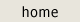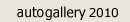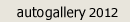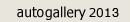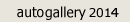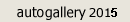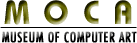Andrew Morse In early 2012, Andrew began coding Ruby plugins for Google SketchUp that produced three- dimensional models based on mathematical functions. His idea was to build visually intriguing models based on equations of three variables, each representing a dimension in space. The same equation was then used to feedback on the initial model and augment its basic shape. This process created stunning, and often unexpected, results that can be seen in each individual piece. Each work of art you see here is based on a mathematical function. Each piece is shown as a 2D representation of a model in 3D space, and each hard-copy print includes a description of the base function that was used as that model's input.